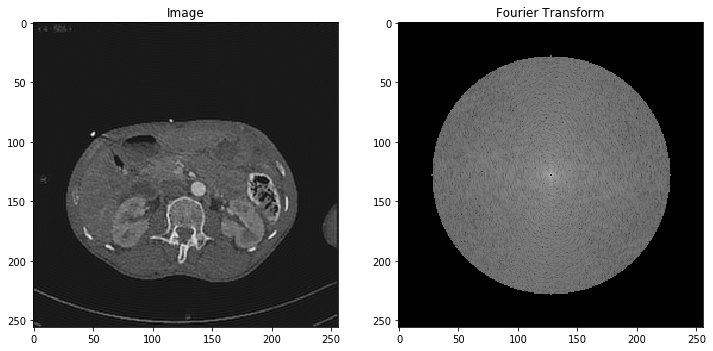# Part 6: Fourier analysis and JPEG compression¶

In the first five notebooks of this workshop session, we've reviewed the fundamentals tools of image processing: numerical arrays, convolutions, point clouds and gradient descent.

Now, for those of you who went really fast through these first steps, here's a little bonus: an introduction to the JPEG (1992) and JPEG2000 compression standards, which are ubiquitous in cameras and cinemas. These two formats rely on the Fourier and Wavelet transforms, two mathematical tools that can be thought of as precursors to Convolutional Neural Networks.

References, going further. These two bonus notebooks are based on the Numerical Tour on approximation with orthogonal bases, written by Gabriel Peyré. For additional references, you may have a look at:

First, we re-import our libraries:

In :
%matplotlib inline
import center_images             # Center our images
import matplotlib.pyplot as plt  # Display library
import numpy as np               # Numerical computations


Re-define custom display routines:

In :
def display(im):  # Define a new Python routine
"""
Displays an image using the methods of the 'matplotlib' library.
"""
plt.figure(figsize=(8,8))                     # Square blackboard
plt.imshow( im, cmap="gray", vmin=0, vmax=1)  # Display 'im' using a gray colormap,
#         from 0 (black) to 1 (white)
def display_2(im_1, title_1, im_2, title_2):
"""
Displays two images side by side; typically, an image and its Fourier transform.
"""
plt.figure(figsize=(12,6))                    # Rectangular blackboard
plt.subplot(1,2,1) ; plt.title(title_1)       # 1x2 waffle plot, 1st cell
plt.imshow(im_1, cmap="gray")                 # Auto-equalization
plt.subplot(1,2,2) ; plt.title(title_2)       # 1x2 waffle plot, 2nd cell
plt.imshow(im_2, cmap="gray", vmin=-7, vmax=15)


And load, once again, a simple axial slice:

In :
I = imread("data/aortes/1.jpg", as_gray=True)  # Import as a grayscale array
I = I / 255                   # Normalize intensities in the [0,1] range
I = I[::2,::2]                # Subsample the image, for convenience
display(I)                    # Let's display our slice: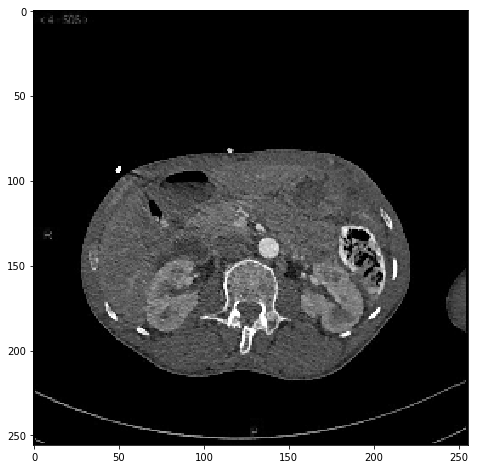### Fourier transform¶

Compression algorithms rely on transforms f, which turn an image I into a new array f(I) that is supposed to be easier to handle. The most fundamental of these "helpers" is the Fourier Transform (click, it's great!), which decomposes a signal or an image as a superposition of harmonics (just like a piano note, really), with weights encoded in the array

fI = fft2(I).
In :
# The numpy packages provides a Fast Fourier Transform in 2D,
# and its inverse (the iFFT). FFTshift and iFFTshift
# are just there to get nicer, centered plots:
from numpy.fft import fft2, ifft2, fftshift, ifftshift

fI = fft2(I)  # Compute the Fourier transform of our slice

# Display the logarithm of the amplitutde of Fourier coefficients.
# The "fftshift" routine allows us to put the zero frequency in
# the middle of the spectrum, thus centering the right plot as expected.
display_2( I, "Image",
fftshift( np.log(1e-7 + abs(fI)) ), "Fourier Transform" )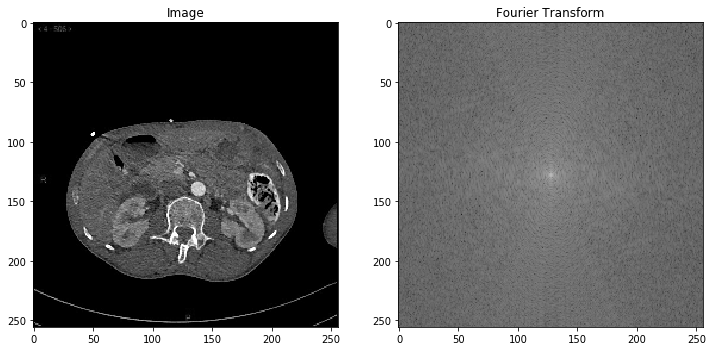To get an intuition of this new object, the simplest thing to do is to take our Fourier Transform fI, edit it, and see what the image ifft2( edit( fI )) looks like:

In :
def Fourier_bandpass(fI, fmin, fmax) :
"""
Truncates a Fourier Transform fI, before reconstructing a bandpassed image.
"""
Y, X = np.mgrid[:fI.shape, :fI.shape]  # Horizontal and vertical gradients
radius = (X - fI.shape/2) ** 2 \
+ (Y - fI.shape/2) ** 2        # Squared distance to the middle point

fI_band = fI.copy()               # Create a copy of the Fourier transform
fI_band[ radius <=fmin ] = 0      # Remove all the low frequencies
fI_band[ radius > fmax ] = 0      # Remove all the high frequencies
I_band = np.real(ifft2(fI_band))  # Invert the new transform...

display_2( I_band, "Image",          # And display
fftshift( np.log(1e-7 + abs(fI_band)) ), "Fourier Transform" )


As evidenced below, Fourier coefficients that are close to the center encode the low frequencies of the signal:

In :
Fourier_bandpass(fI, 0, 10)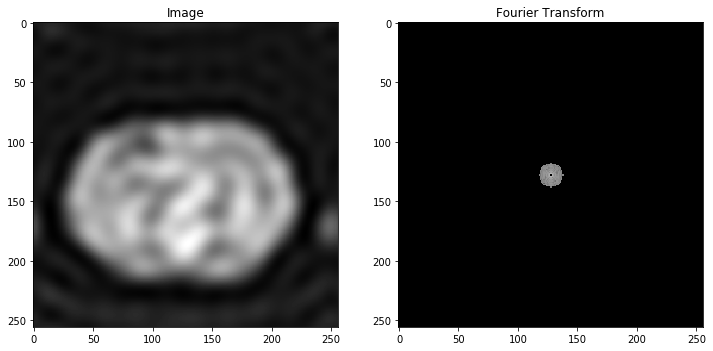As we add more coefficients, we see that details start to appear:

In :
Fourier_bandpass(fI, 0, 50)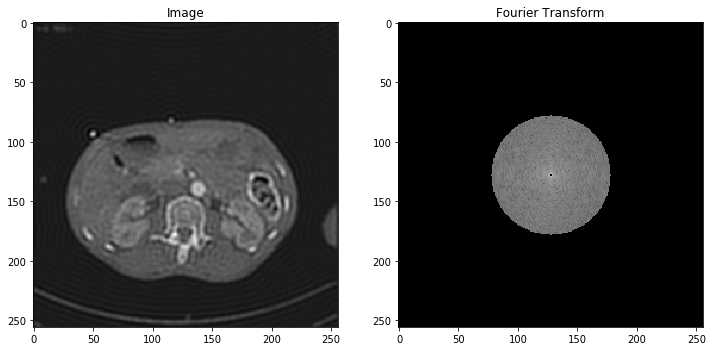We can also keep specific frequencies and compute "detail-only" images at a cheap numerical cost. Convolutions, that we presented in the 2nd notebook, can all be implemented this way:

In :
Fourier_bandpass(fI, 50, 100)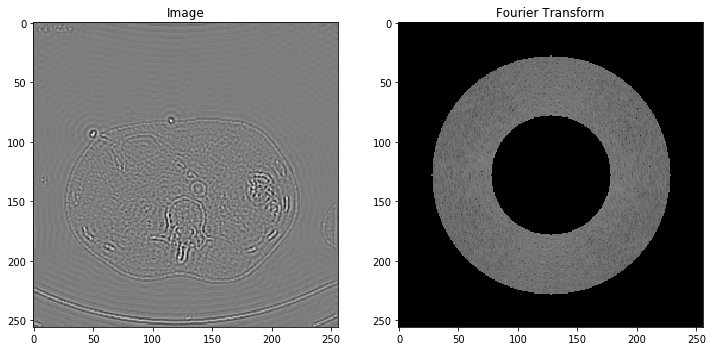Our image can then be simply expressed as a sum of low, medium and high frequencies:

In :
Fourier_bandpass(fI, 0, 100)  # = Sum of the last two images